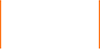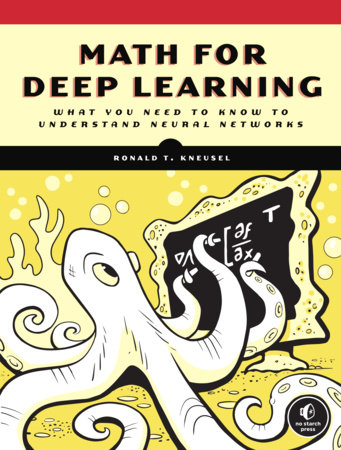# Math for Deep Learning

Best SellerPaperback \$49.99
Dec 07, 2021 | ISBN 9781718501904

#### Paperback –

• Dec 07, 2021 | ISBN 9781718501904

#### Ebook +

• Nov 23, 2021 | ISBN 9781718501911

#### Praise

“An excellent resource for anyone looking to gain a solid foundation in the mathematics underlying deep learning algorithms. The book is accessible, well-organized, and provides clear explanations and practical examples of key mathematical concepts. I highly recommend it to anyone interested in this field.”
—Daniel Gutierrez, insideBIGDATA

“Ronald T. Kneusel has written a handy and compact guide to the mathematics of deep learning. It will be a well-worn reference for equations and algorithms for the student, scientist, and practitioner of neural networks and machine learning. Complete with equations, figures and even sample code in Python, this book is a wonderful mathematical introduction for the reader.”
—David S. Mazel, Senior Engineer, Regulus-Group

“What makes Math for Deep Learning a stand-out, is that it focuses on providing a sufficient mathematical foundation for deep learning, rather than attempting to cover all of deep learning, and introduce the needed math along the way. Those eager to master deep learning are sure to benefit from this foundation-before-house approach.”
Ed Scott, Ph.D., Solutions Architect & IT Enthusiast

Introduction
Chapter 1: Setting the Stage
Chapter 2: Probability
Chapter 3: More Probability
Chapter 4: Statistics
Chapter 5: Linear Algebra
Chapter 6: More Linear Algebra
Chapter 7: Differential Calculus
Chapter 8: Matrix Calculus
Chapter 9: Data Flow in Neural Networks
Chapter 10: Backpropagation